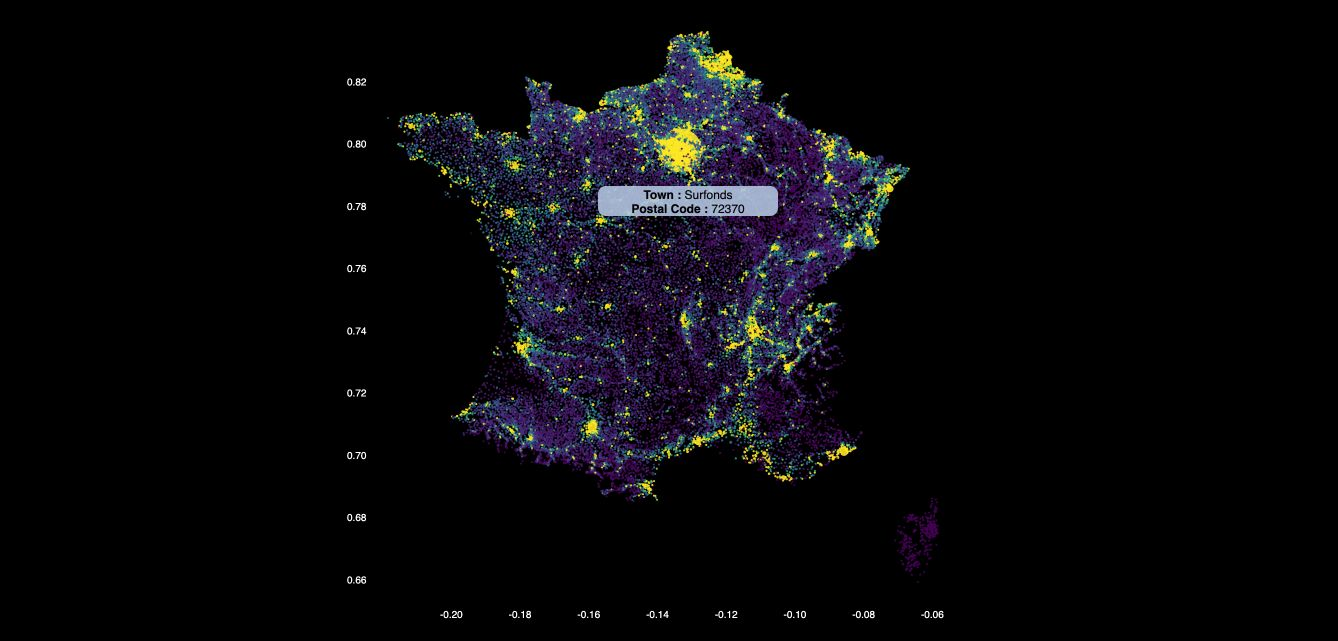# Perceptron

Let $$(x_1, y_1), ..., (x_n, y_n) \in R^d \times \{ ±1 \}$$ be labeled training data. The data is said to be linearly separable if there exists a hyperplane that correctly classifies all the examples :

$\forall t \in n, y_t \langle w^*, x_t \rangle > 0$

In general, finding $$w^*$$ is impossible, but we search for some $$\hat{w}$$ that separates the 2 classes. The objective function to optimize is :

$f(w) = \sum_t 1(y_t \langle w^*, x_t \rangle ≥ 0)$

This is called a batch objective since it relies on a cumulative fit to data. By our assumption : $$f(w^*) = 0$$. There are however many solution hyperplanes if we consider scaling of $$w^*$$. To solve this, we fix $$w^*$$ to be the smallest-norm vector that guarantees :

$\forall t \in n, y_t \langle w^*, x_t \rangle ≥ 1$

How can we solve this? Using the Perceptron recursive update. It has been shown that the perceptron converges to a solution in a finite number of steps.

The algorithm of the Perceptron is the following :Conclusion : That’s it ! I hope this introduction to Online Learning was clear. Don’t hesitate to drop a comment if you have any question.

Categories:

Updated: